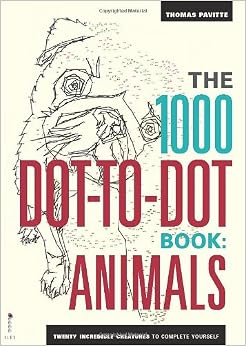### February 2016

Do you remember free time as a kid that you wasted with connecting dots? If you actually liked it, here’s some good news: There are dot-to-dot books for grown-ups! Most notable, there are the books by Thomas Pavitte with great pictures with 1000 dots.

So, these are some of the booksand here is some video:

Actually, it takes some time to connect 1000 dots; I need ten minutes or so, depending a bit an the picture.

For the fun of it, I coded some lines in MATLAB to connect the dots automatically. And since I am a lazy programmer, I did not bother to connect the dots in the manner that was prescribed by the artist but more efficiently:

1. Greedy paths

For the greedy path, we start at some randomly chosen dot and connect the dot where we are with the closest possible dot where we haven’t been already.

Here’s how this looks for one of Thomas’ pictures:2. Shortest paths

The greedy path sometimes makes very large jumps (when it runs into some corner, using all the dots in the vicinity). This leads to some spurious large jumps in the picture. In used some simple heuristics to find some “locally shortest paths” through the thousand dots. (And “locally shortest” means that there are no two edges for which swapping improves the total lengths of the paths.) Actually, I started out with the goal to solve the travelling salesman problem over the thousand dots, i.e., to find the shortest path of all. Then it turned out that

1. Solving the travelling salesman problem is not that simple to solve – well it’s one of the best investigated NP-hard optimization problems and there is no doubt that it would take my laptop only little time to solve it with 1000 dots if fed with the right code.
2. The locally shortest path already looks quite appealing and I am not sure how the shortest path would look any different.

Here is the locally shortest path:Oh, by the way: the image is a pug dog, the one that you can party see on this coverHere are some more pictures (not by Thomas Pavitte). Middle is the greedy, right the locally shortest path:

This slideshow requires JavaScript.

Since the Wikipedia page of the travelling salesman problem contains a formulation as an integer linear program to solve it, I may give it a try in the future…

Im Wintersemester 2015/2016 habe ich die Vorlesung “Analysis 3” gehalten und dazu dieses Skript verfasst:

Diese Seite dient dazu, in den Kommentaren gefundene Fehler zu sammlen und hier zu dokumentieren.

Errata zur gedruckten Version:

• Seite 16: “$|\det(U)|$ und$\int_{\mathbb{R}}$ statt$\int_{\mathbb{-R}}$
• Seite 17, zweiter Absatz: “wobei$G$ eine Teilmenge des$\mathbb{R}^{n+1}$ ist”
• Seite 34 Beispiel 18.5 ii) Argument leicht umformuliert da vorher nicht ganz korrekt
• Seite 45:$\varphi_2 = \dots = \varphi''$
• Seite 58 Satz 19.3 Schritt2. die letzte Zeile. ” Dies heißt aber, dass$\varphi^1,\dots,\varphi^n$ linear abhängig sind. Widerspruch!”
Korrektur: “Dies heißt aber, dass$\psi^1,\dots,\psi^n$ linear abhängig sind. Widerspruch!”
• Seite 59 die erste Bemerkung (zweite Zeile): Lösungen$\varphi^k$ statt$\psi^k$;
• Seite 61 Korollar 19.7 “Fundamentalmatrix zu$y'=A(x)y$” statt “$y'=A(x)$“.
• Seite 70, Beweis von Satz 19.17:$+b(x)$ statt$-b(x)$.
• Seite 80, Beipsiel 19.29, 2.:$\mathrm{i}\omega$ ist eine Nullstelle.
• Seite 107, Satz 21.4:$\tilde\phi:\tilde T\to M$.
• Seite 116:$\omega_5 = \tfrac{8\pi^2}{3}$.
• Seite 137 unten: “aus$f_\nu\to f$ glm. folgt…”
• Seite 140, Beweis von Lemma 23.11:$\partial^\alpha\phi_\nu\stackrel{\mathcal{D}}{\to}\partial^\alpha\phi$ .
• Seite 141:$[x\phi(x)]_{-\infty}^0$
• Seite 152:$(LE)*\rho = \delta_0*\rho$

Das Skript zur zugehörigen Vorlesung “Analysis 2” ist hier zu finden.